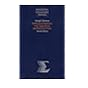Normal view

# Differential equations, with applications and historical notes / George F. Simmons, with a new chapter on numerical methods by John S. Robertson.

Material type:TextLanguage: English Publisher: New York : McGraw-Hill, c1991[reprint 2003]Edition: 2nd edDescription: xxi, 629 p. : ill. ; 24 cmISBN: 0070575401 :; 9780070575400DDC classification: 515.35 LOC classification: QA372 | .S49 1991Online resources: Publisher description | Table of contents | WorldCat details
Contents:
Table of contents 1. The nature of differential equations -- Families of curves -- Orthogonal trajectories -- Growth, decay, chemical reactions, and mixing -- Falling bodies and other motion problems -- The Brachistochrone -- Fermat and the Bernoullis -- 2. First order equations -- Homogeneous equations -- Exact equations -- Integrating factors -- Linear equations -- Reduction of order -- The hanging chain -- Pursuit curves -- Simple electric circuits -- 3. Second order linear equations -- Vibrations in mechanical and electrical systems -- Newton's Law of Gravitation and the motion of the planets -- Coupled harmonic oscillators -- 4. Qualitative properties of solutions -- Oscillations and the Sturm Separation theorem -- The Sturm Comparison theorem -- 5. Power series solutions and special functions -- Gauss's hypergeometric equation -- The point at infinity -- Hermite polynomials and quantum mechanics -- Chebyshev polynomials and the minimax property -- Riemann's equation -- 6. Fourier series and orthogonal functions -- 7. Partial differential equations and boundary value problems -- Eigenvalues, eigenfunctions, and the vibrating string -- The heat equation -- The Dirichlet problem for a circle -- Poisson's integral -- Sturm-Liouville problems -- 8. Some special functions of mathematical physics -- Legendre polynomials -- Bessel functions -- 9. Laplace transforms -- 10. Systems of first order equations -- Linear systems -- 11. Linear equations -- Liapunov -- Poincccaré-Bendixson theorem -- Proof of Liénard's theorem -- 12. The calculus of variations -- 13. The existence and uniqueness of solutions -- Successive approximations -- Picard -- 14. Numerical methods -- Euler.
Tags from this library: No tags from this library for this title.Average rating: 0.0 (0 votes)
Item type Current location Collection Call number Copy number Status Date due Barcode Item holdsText
Reserve Section
Non-fiction 515.35 SID 1991 (Browse shelf) C-1 Not For Loan 13662Text
Reserve Section
Non-fiction 515.35 SID 1991 (Browse shelf) C-2 Not For Loan 13993Text
Reserve Section
Non-fiction 515.35 SID 1991 (Browse shelf) C-3 Not For Loan 13994Text
Circulation Section
Non-fiction 515.35 SID 1991 (Browse shelf) C-4 Available 13995Text
Circulation Section
Non-fiction 515.35 SID 1991 (Browse shelf) C-5 Available 13996Text
Circulation Section
Non-fiction 515.35 SID 1991 (Browse shelf) C-6 Available 14122
Total holds: 0
##### Browsing EWU Library shelves, Shelving location: Reserve Section Close shelf browser515.35 ROD 1984 Differential equations / 515.35 SID 1991 Differential equations, with applications and historical notes / 515.35 SID 1991 Differential equations, with applications and historical notes / 515.35 SID 1991 Differential equations, with applications and historical notes / 515.35 TRE 2001 Elementary differential equations with boundary value problems / 515.35 ZIF 2001 A first course in differential equations with modeling applications / 515.35 ZIF 2005 A first course in differential equations with modeling applications /

Includes bibliographical references and index.

Table of contents 1. The nature of differential equations --
Families of curves --
Orthogonal trajectories --
Growth, decay, chemical reactions, and mixing --
Falling bodies and other motion problems --
The Brachistochrone --
Fermat and the Bernoullis --
2. First order equations --
Homogeneous equations --
Exact equations --
Integrating factors --
Linear equations --
Reduction of order --
The hanging chain --
Pursuit curves --
Simple electric circuits --
3. Second order linear equations --
Vibrations in mechanical and electrical systems --
Newton's Law of Gravitation and the motion of the planets --
Coupled harmonic oscillators --
4. Qualitative properties of solutions --
Oscillations and the Sturm Separation theorem --
The Sturm Comparison theorem --
5. Power series solutions and special functions --
Gauss's hypergeometric equation --
The point at infinity --
Hermite polynomials and quantum mechanics --
Chebyshev polynomials and the minimax property --
Riemann's equation --
6. Fourier series and orthogonal functions --
7. Partial differential equations and boundary value problems --
Eigenvalues, eigenfunctions, and the vibrating string --
The heat equation --
The Dirichlet problem for a circle --
Poisson's integral --
Sturm-Liouville problems --
8. Some special functions of mathematical physics --
Legendre polynomials --
Bessel functions --
9. Laplace transforms --
10. Systems of first order equations --
Linear systems --
11. Linear equations --
Liapunov --
Poincccaré-Bendixson theorem --
Proof of Liénard's theorem --
12. The calculus of variations --
13. The existence and uniqueness of solutions --
Successive approximations --
Picard --
14. Numerical methods --
Euler.

Electrical & Electronic Engineering

There are no comments for this item.

to post a comment.
Share

Library Home | Contacts | E-journals
Copyright @ 2011-2019 EWU Library
East West University